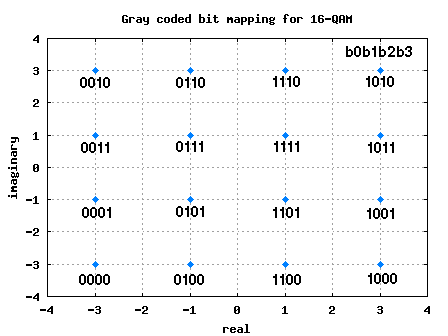- DSP log - http://www.dsplog.com -

Binary to Gray code for 16QAM

Posted By Krishna Sankar On June 1, 2008 @ 6:28 am In Modulation | 13 Comments

In the previous post on Binary to Gray code conversion for PSK, I had claimed that “for a general M-QAM modulation the binary to Gray code conversion is bit more complicated“. However following a closer look, I realize that this is not so complicated.The QAM scenario can be treated as independent PAM modulation on I arm and Q-arm respectively. For example, let us consider 16-QAM scenario.

Each constellation point can represent bits, with two bits on the I axis and two on the Q axis. For 16-QAM, the values taken by the I and Q axes are {-3, -1, +1, +3}. The two bits on the I and Q arm can be Gray coded as shown in the table below

 b0b1 I b2b3 Q 00 -3 00 -3 01 -1 01 -1 11 +1 11 +1 10 +3 10 +3

Table: Gray coded constellation mapping for 16-QAM

The constellation diagram with the bit mapping is shown below.Figure: 16QAM constellation with Gray coded mapping

As can be observed from the figure above, the adjacent constellation symbols differ by only one bit. As simple as that.Simulation model for Binary to Gray coded mapping for 16-QAM

% Matlab/Octave code for converting bits into 16-QAM constellation

clear all
M = 16;
% number of constellation points
k = log2(M); % number of bits in each constellation
% defining the real and imaginary PAM constellation
% for 16-QAM
alphaRe = [-(2*sqrt(M)/2-1):2:-1 1:2:2*sqrt(M)/2-1];
alphaIm = [-(2*sqrt(M)/2-1):2:-1 1:2:2*sqrt(M)/2-1];
% input - decimal equivalent of all combinations with b0b1b2b3
ip = [0:15];
ipBin = dec2bin(ip.'); % decimal to binary
% taking b0b1 for real
ipDecRe = bin2dec(ipBin(:,[1:k/2]));
ipGrayDecRe = bitxor(ipDecRe,floor(ipDecRe/2));
% taking b2b3 for imaginary
ipDecIm = bin2dec(ipBin(:,[k/2+1:k]));
ipGrayDecIm = bitxor(ipDecIm,floor(ipDecIm/2));
% mapping the Gray coded symbols into constellation
modRe = alphaRe(ipGrayDecRe+1);
modIm = alphaIm(ipGrayDecIm+1);
% complex constellation
mod = modRe + j*modIm;

Note:

The above code snippet works only for 4QAM and 16QAM.